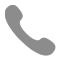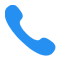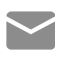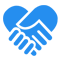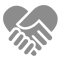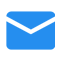# 三大深度学习生成模型：VAE、GAN及其变种

2017.08.09

### VAEVariational Lower bound• 右边第一项的log似然的期望最大化：• 右边第二项的KL散度最小化：Reparameterization TrickEncoder和Decoder的计算公式• Encoder的Loss计算：KL散度。

• z的重采样生成。

• Decoder的Loss计算：最大似然。MNIST生成模型可视化

1. 隐变量的维度为2，相当于把生成的数字图片投影到2维平面上，这样更方便可视化观察分析。

2. 由于隐变量的维度为2，就可以从二维平面上等间距地采样一批隐变量，这样这批隐变量可以代表整个二维平面上隐变量的分布，然后这批隐变量经过解码器处理后展示，这样就可以看到图像的分布情况了。VAE的内容就介绍到这里，下面来看看另一个生成模型。

### GAN

GAN的概念

• 判别模型的目标是最大化这个公式：Ex[D(x)]，也就是甄别出哪些图是真实数据分布中的。

• 生成模型的目标是最大化这个公式：Ez[D(G(z))]，也就是让自己生成的图被判别模型判断为来自真实数据分布。`def GAN(G,D,X):    # G 表示生成模型    # D 表示判别模型    # X 表示训练数据    for iter in range(MAX_ITER):        for step in range(K):            x = data_sample(X)            z = noise_sample()            optimize_D(G, D, x, z)        z = noise_sample()        optimize_G(G, D, z)`

GAN的训练分析`import numpy as npimport mathdef KL(p, q):    # p,q为两个list，里面存着对应的取值的概率，整个list相加为1    if 0 in q:        raise ValueError    return sum(_p * math.log(_p/_q) for (_p,_q) in zip(p, q) if _p != 0)def JS(p, q):    M = [0.5 * (_p + _q) for (_p, _q) in zip(p, q)]    return 0.5 * (KL(p, M) + KL(q, M))`

`def exp(a, b):    a = np.array(a, dtype=np.float32)    b = np.array(b, dtype=np.float32)    a /= a.sum()    b /= b.sum()    print a    print b    print KL(a,b)    print JS(a,b)# exp 1exp([1,2,3,4,5],[5,4,3,2,1])#以下为运行结果显示[ 0.066  0.133  0.2         0.266  0.333][ 0.333  0.266  0.2         0.133  0.066]0.5210.119`

`# exp 2exp([1,2,3,4,5],[1e-12,4,3,2,1])exp([1,2,3,4,5],[5,4,3,2,1e-12])#以下为运行结果显示[ 0.066  0.133  0.2         0.266  0.333][ 9.999e-14   4.000e-01   3.000e-01   2.000e-01   1.000e-01]2.065502018460.0985487692551[ 0.066  0.133  0.2         0.266  0.333][ 3.571e-01   2.857e-01   2.142e-01   1.428e-01   7.142e-14]9.6620.193`

`# exp 3exp([1e-12,2,3,4,5],[5,4,3,2,1])exp([1,2,3,4,1e-12],[5,4,3,2,1])`

`[ 7.142e-14   1.428e-01   2.142e-01   2.857e-01   3.571e-01][ 0.333  0.266  0.2         0.133  0.0666]0.7420.193[ 1.000e-01   2.000e-01   3.000e-01   4.000e-01   9.999e-14][ 0.333  0.266  0.2         0.133  0.066]0.3830.098`

GAN实战• 将Pooling层替换成带有stride的卷积层

• 使用Batch Normalization

• 放弃使用全连接层

• 将卷积层的非线性部分换成ReLU或者Leaky ReLU

`def generator_model():    model = Sequential()    model.add(Dense(input_dim=100, output_dim=1024))    model.add(Activation('tanh'))    model.add(Dense(out_dim=128*7*7))    model.add(BatchNormalization())    model.add(Activation('tanh'))    model.add(Reshape((128, 7, 7), input_shape=(128*7*7,)))    model.add(UpSampling2D(size=(2, 2)))    model.add(Convolution2D(out_channel=64, kernel_height=5, kernel_width=5, border_mode='same'))    model.add(Activation('tanh'))    model.add(UpSampling2D(size=(2, 2)))    model.add(Convolution2D(out_channel=1, kernel_height=5, kernel_width=5, border_mode='same'))    model.add(Activation('tanh'))    return model`

`def discriminator_model():    model = Sequential()    model.add(Convolution2D(64, 5, 5, border_mode='same', input_shape=(1, 28, 28)))    model.add(Activation('tanh'))    model.add(MaxPooling2D(pool_size=(2, 2)))    model.add(Convolution2D(128, 5, 5))    model.add(Activation('tanh'))    model.add(MaxPooling2D(pool_size=(2, 2)))    model.add(Flatten())    model.add(Dense(1024))    model.add(Activation('tanh'))    model.add(Dense(1))    model.add(Activation('sigmoid'))    return model`20轮Epoch后的结果如图10-11所示。### Info-GAN`import numpy as npimport mathdef mutual_info(x_var, y_var):    sum = 0.0    x_set = set(x_var)    y_set = set(y_var)    for x_val in x_set:        px = float(np.sum(x_var == x_val)) / x_var.size        x_idx = np.where(x_var == x_val)        for y_val in y_set:            py = float(np.sum(y_var == y_val)) / y_var.size            y_idx = np.where(y_var == y_val)            pxy = float(np.intersect1d(x_idx, y_idx).size) / x_var.size            if pxy > 0.0:                sum += pxy * math.log((pxy / (px * py)), 10)    return sum`

`a = np.array([0,0,5,6,0,4,4,3,1,2])b = np.array([3,4,5,5,3,7,7,6,5,1])print mutual_info(a,b)# 0.653a = np.array([0,0,5,6,0,4,4,3,1,2])b = np.array([3,3,5,6,3,7,7,9,4,8])print mutual_info(a,b)# 0.796`

“身体和灵魂，总有一个在路上”，是不是标榜追求最大熵的一个口号？“公务员这种稳定工作才是好工作”是不是一种追求最小化熵的行为呢？那么对于一个人来说，究竟是熵越大越好，还是熵越小越好？

InfoGAN模型

• I(X;Z)=H(X)-H(X|Z)

• I(X;Z)=H(Z)-H(Z|X)• reg_z：表示了模型开始随机生成的隐变量。

• fake_ref_z_dist_info：表示了经过Encoder计算后的隐变量分布信息。

• cont_reg_z：reg_z的连续变量部分

• cont_reg_dist_info：fake_ref_z_dist_info的连续变量部分

• disc_reg_z：reg_z的离散变量部分

• disc_reg_dist_info：fake_ref_z_dist_info的连续变量部分

• 接下来，四个变量两两组队完成了后验公式P(c)logQ(c|g?([c,z])的计算：

• cont_log_q_c_given_x：连续变量的后验

• disc_log_q_c_given_x：离散变量的后验

• cont_log_q_c：连续变量的先验

• disc_log_q_c：离散变量的后验

• cont_cross_ent：连续变量的交叉熵

• cont_ent：连续变量的熵

• disc_cross_ent：离散变量的交叉熵

• disc_ent：离散变量的熵

• cont_mi_est：连续变量的互信息

• disc_mi_est：离散变量的互信息### 总结

• VAE：基于变分下界约束得到的Encoder-Decoder模型对。

• GAN：基于对抗的Generator-Discriminator模型对。

• InfoGAN：挖掘GAN模型隐变量特点的模型。

（转载自：http://geek.csdn.net/news/detail/230599）